# How to Calculate and Solve for Volume Ratio | Design of Gating System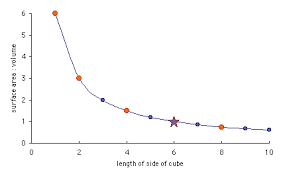The image above represents volume ratio.

To compute for volume ratio, two essential parameters are needed and these parameters are Mass of the Liquid Metal filling the Mould Cavity, Gates and Risers (G) and Casting Volume (V).

The formula for calculating volume ratio:

Kv = G / V

Where:

Kv = Volume Ratio
G = Mass of the Liquid Metal Filling the Mould Cavity,Gates and Risers
V = Casting Volume

Let’s solve an example;
Find the volume ratio when the mass of the liquid metal filling the mould cavity, gates and risers is 12 and the casting volume is 24.

This implies that;

G = Mass of the Liquid Metal Filling the Mould Cavity,Gates and Risers = 12
V = Casting Volume  = 24

Kv = G / V
Kv = 12 / 24
Kv = 0.5

Therefore, the volume ratio is 0.5.

Calculating the Mass of the Liquid Metal Filling the Mould Cavity, Gates and Risers when the Volume Ratio and the Casting Volume is Given.

G = Kv x V

Where;

G = Mass of the Liquid Metal Filling the Mould Cavity,Gates and Risers
Kv = Volume Ratio
V = Casting Volume

Let’s solve an example;
Find the mass of the liquid metal filling the mould cavity, gates and risers when the volume ratio is 15 and the casting volume is 10.

This implies that;

Kv = Volume Ratio = 15
V = Casting Volume = 10

G = Kv x V
G = 15 x 10
G = 150

Therefore, the mass of the liquid metal filling the mould cavity,gates and risers is 150.

Calculating the Casting Volume when the Volume Ratio and the Mass of the Liquid Metal Filling the Mould Cavity, Gates and Risers is Given.

V = Kv / G

Where;

V = Casting Volume
Kv = Volume Ratio
G = Mass of the Liquid Metal Filling the Mould Cavity,Gates and Risers

Let’s solve an example;
Find the casting volume when the volume ratio is 62 and the mass of the liquid metal filling the mould cavity,gates and risers is 22.

This implies that;

Kv = Volume Ratio = 62
G = Mass of the Liquid Metal Filling the Mould Cavity,Gates and Risers = 22

V = Kv / G
V = 62 / 22
V = 2.81

Therefore, the casting volume is 2.81.

Nickzom Calculator – The Calculator Encyclopedia is capable of calculating the volume ratio.

To get the answer and workings of the volume ratio using the Nickzom Calculator – The Calculator Encyclopedia. First, you need to obtain the app.

You can get this app via any of these means:

To get access to the professional version via web, you need to register and subscribe for NGN 1,500 per annum to have utter access to all functionalities.
You can also try the demo version via https://www.nickzom.org/calculator

Apple (Paid) – https://itunes.apple.com/us/app/nickzom-calculator/id1331162702?mt=8
Once, you have obtained the calculator encyclopedia app, proceed to the Calculator Map, then click on Materials & Metallurgical under Engineering.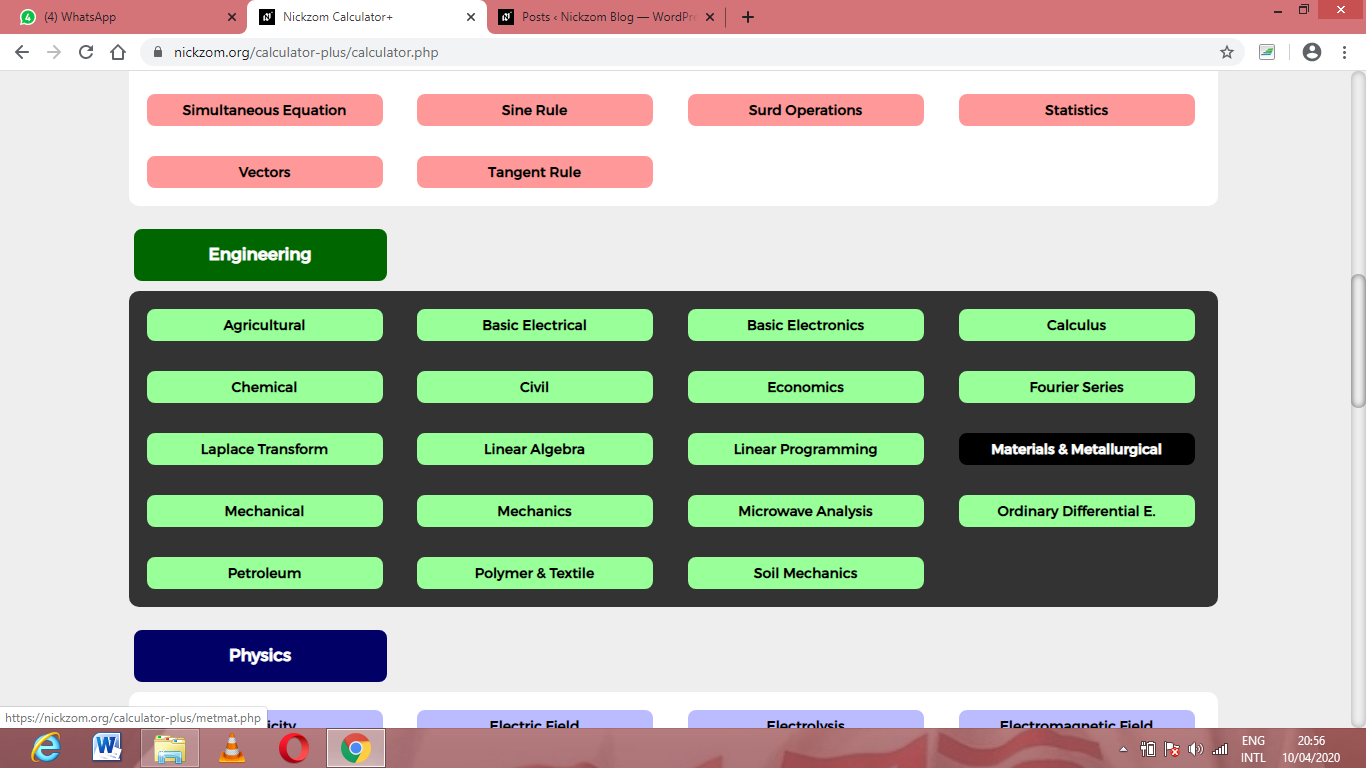Now, Click on Foundry Technology under Material & Metallurgical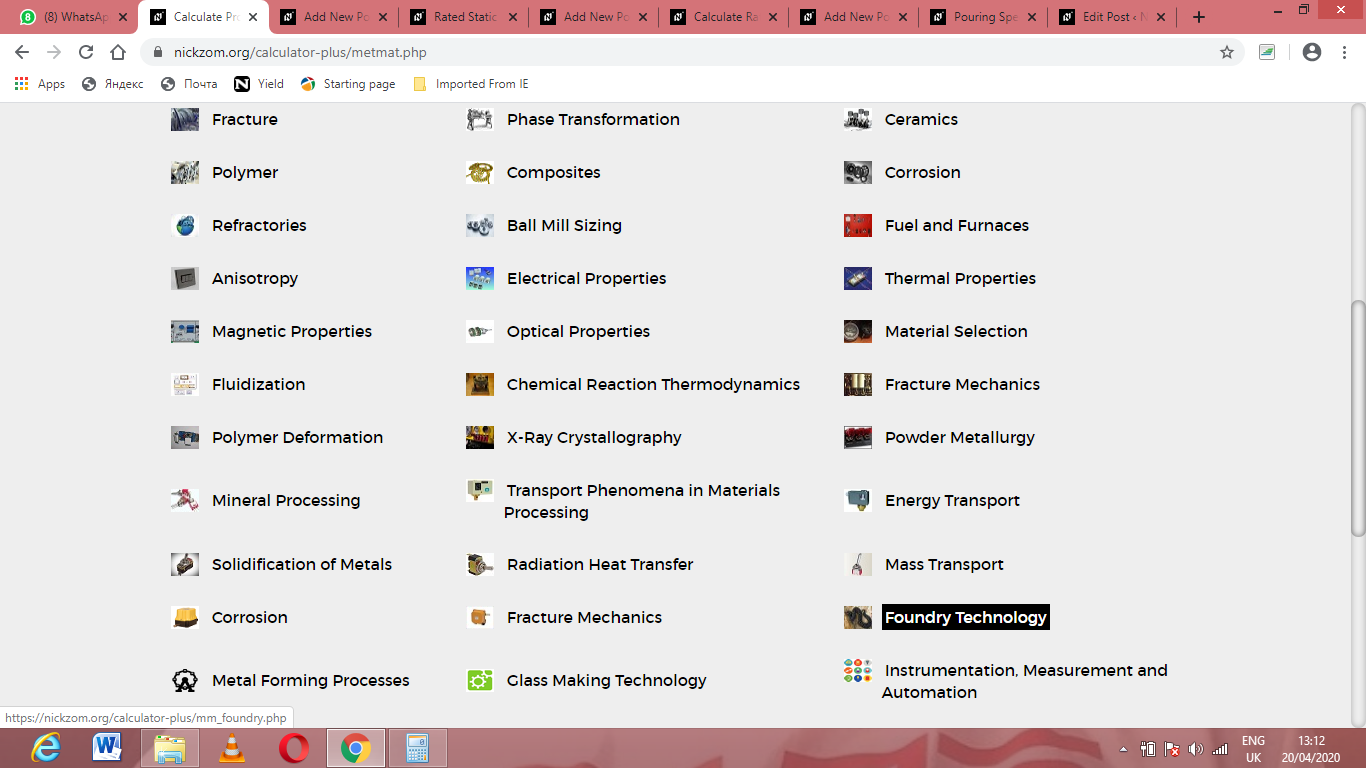Now, Click on Design of Gating System under Foundry Technology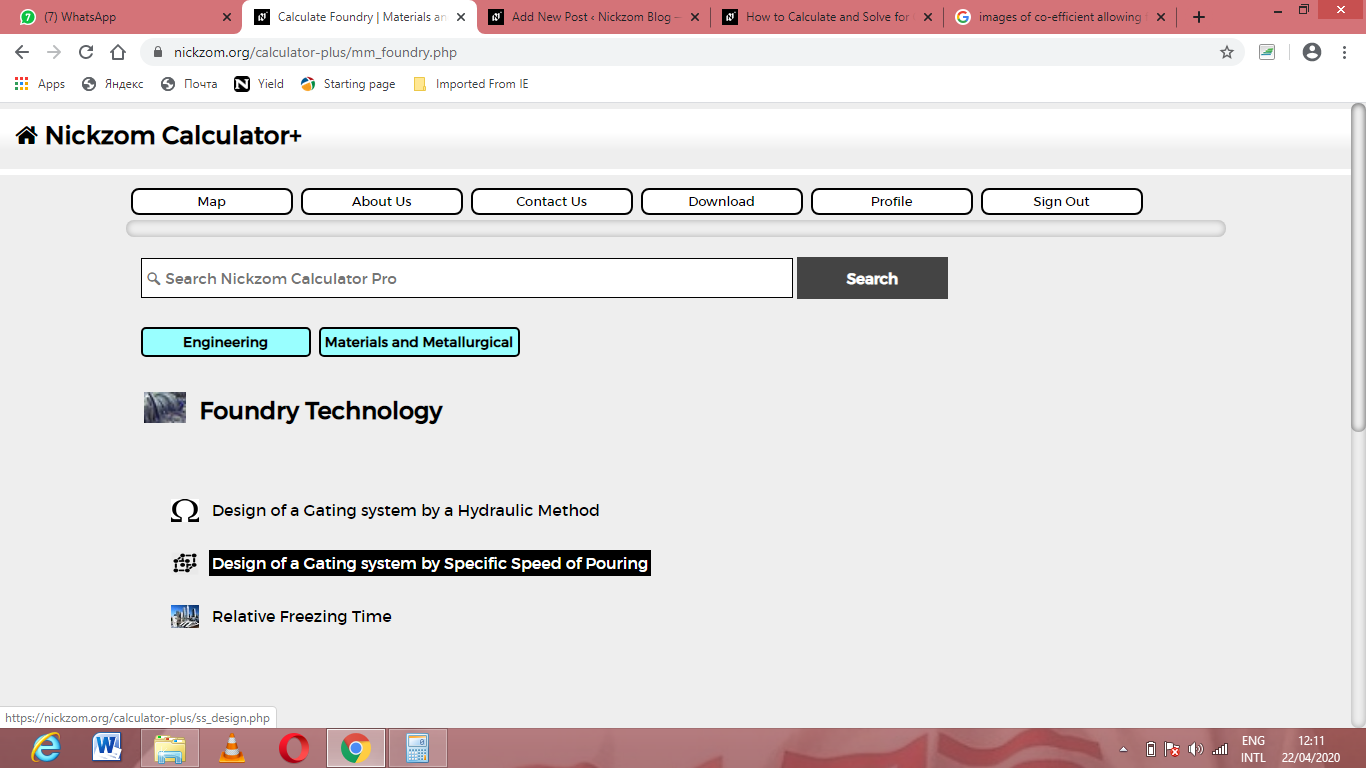Now, Click on Volume Ratio under Design of Gating System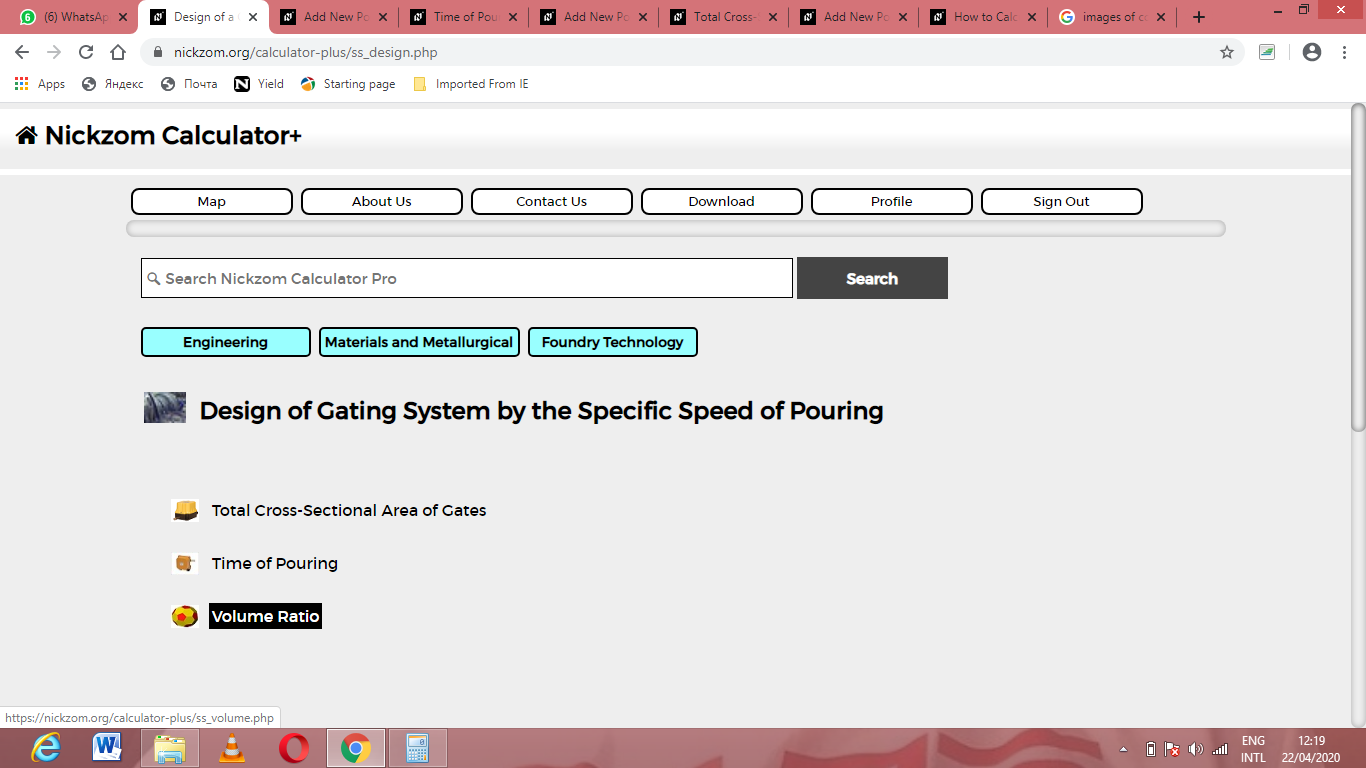The screenshot below displays the page or activity to enter your values, to get the answer for the volume ratio according to the respective parameters which are the Mass of the Liquid Metal filling the Mould Cavity, Gates and Risers (G) and Casting Volume (V).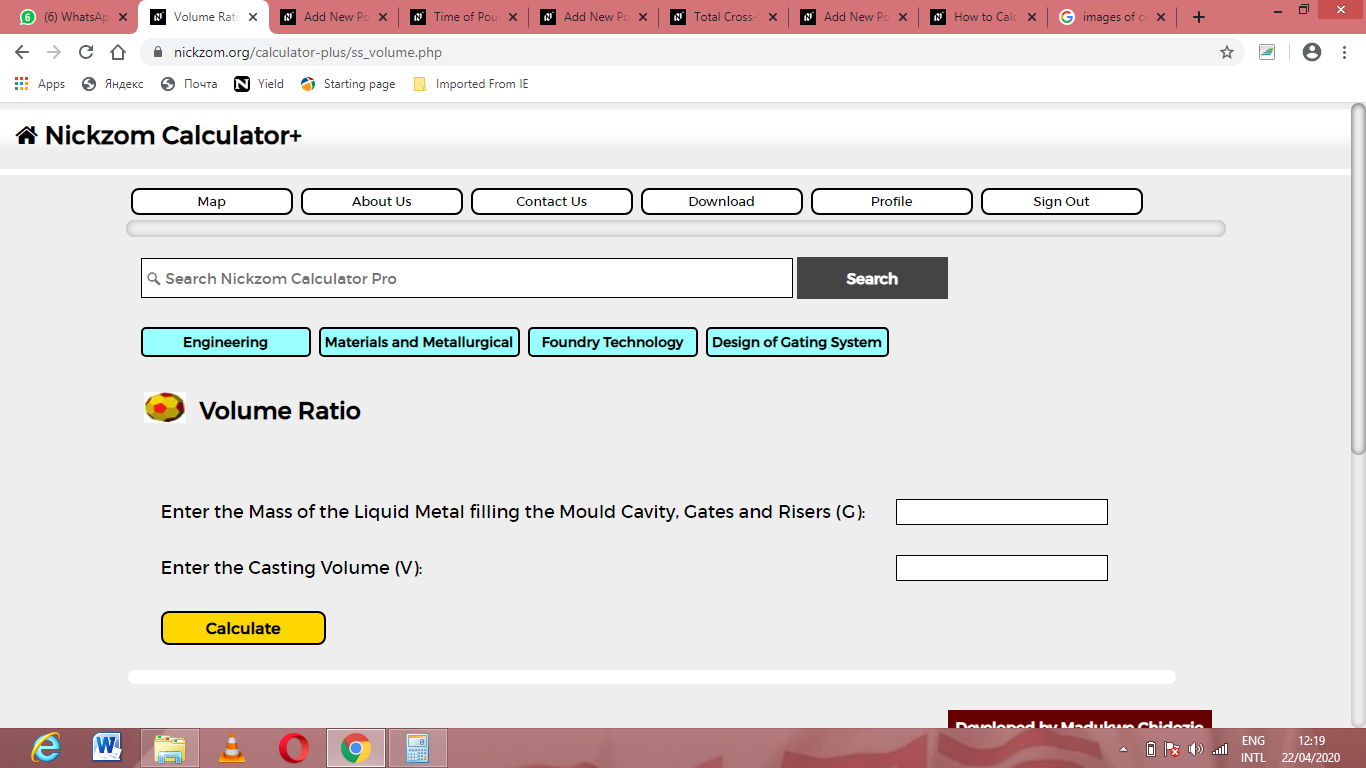Now, enter the values appropriately and accordingly for the parameters as required by the Mass of the Liquid Metal filling the Mould Cavity, Gates and Risers (G) is 12 and Casting Volume (V) is 24.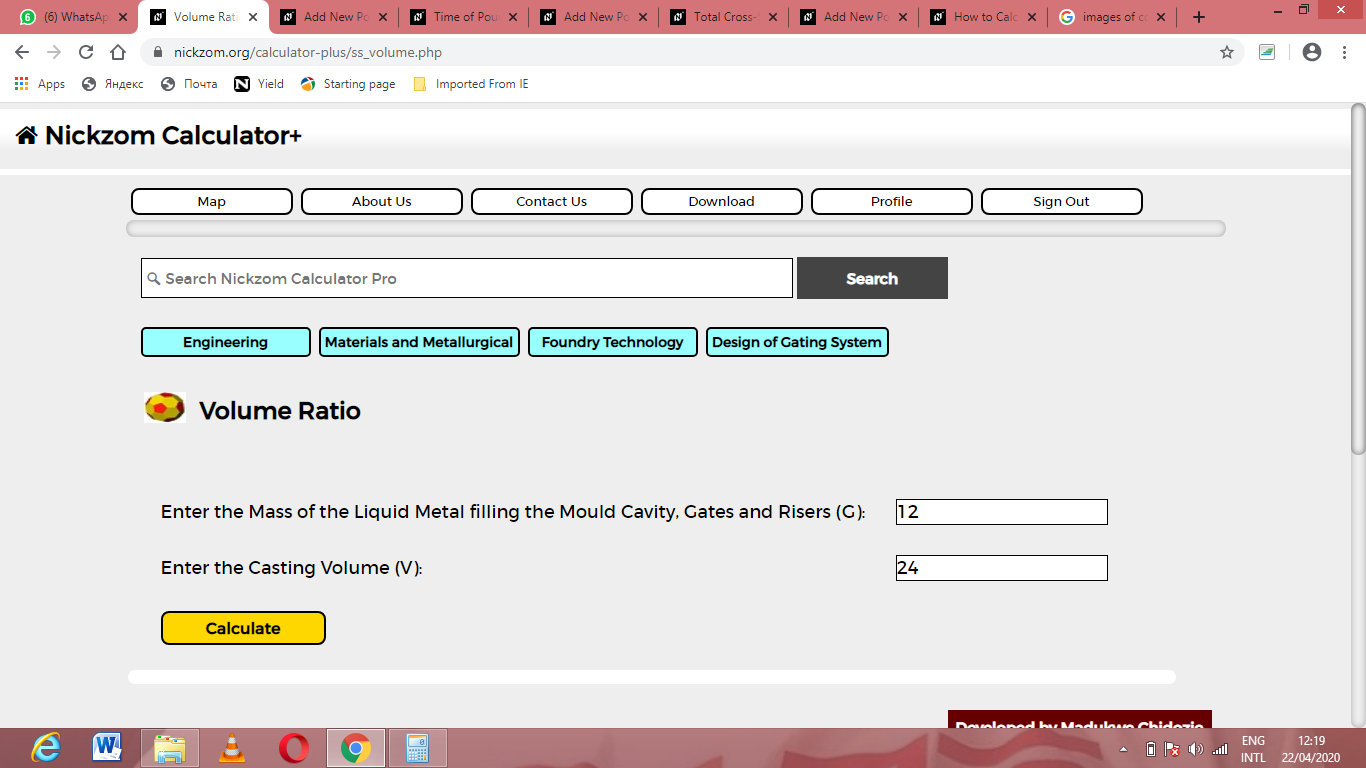Finally, Click on Calculate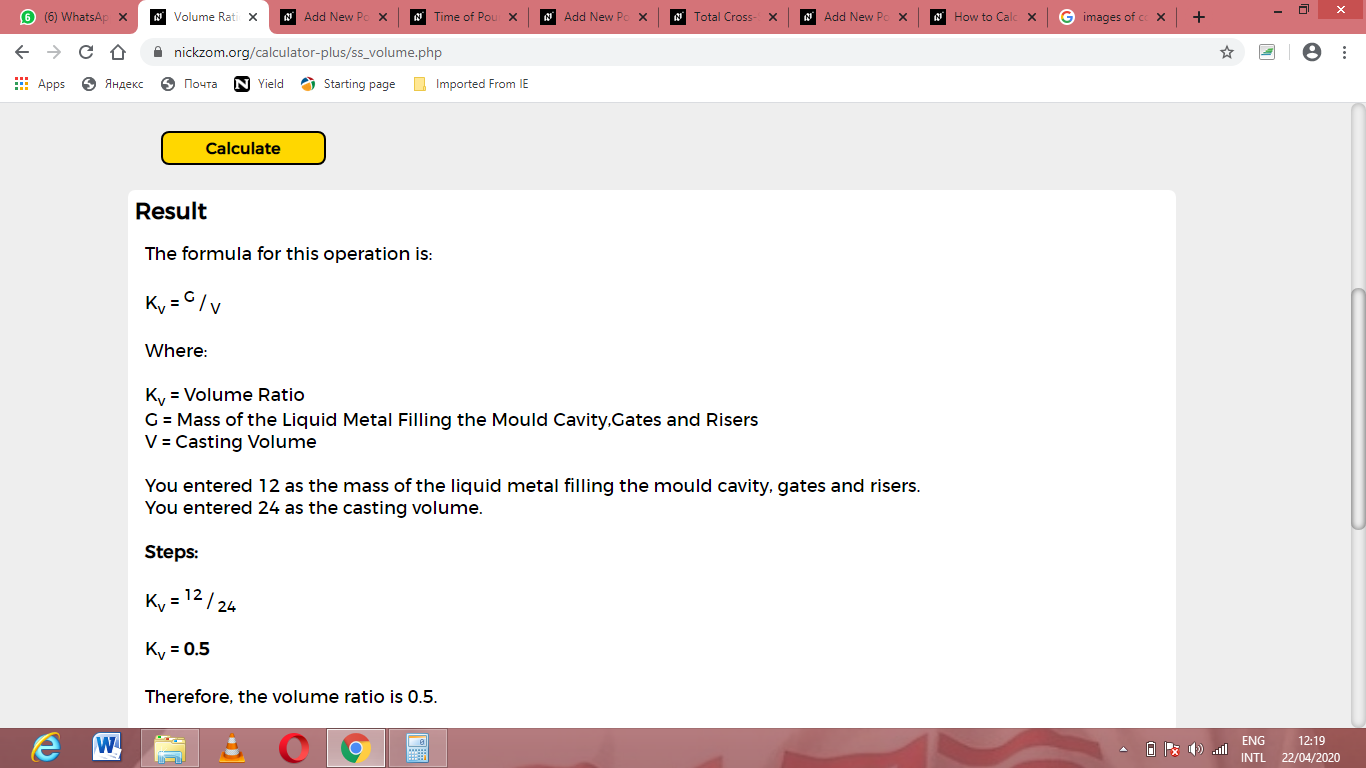As you can see from the screenshot above, Nickzom Calculator– The Calculator Encyclopedia solves for the volume ratio and presents the formula, workings and steps too.